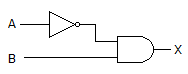# Digital Electronics - Logic Gates - Discussion

### Discussion :: Logic Gates - Filling the Blanks (Q.No.1)

1.

The gates in this figure are implemented using TTL logic. If the input of the inverter is open, and you apply logic pulses to point B, the output of the AND gate will be ________.[A]. a steady LOW [B]. a steady HIGH [C]. an undefined level [D]. pulses

Explanation:

No answer description available for this question.

 Gopi said: (Aug 1, 2011) It is a wrong answer because 1*pulses gives the pulses output.

 Sachin Dohre said: (Oct 1, 2012) If A is open means undefined so output must be undefined level.

 Nandi said: (Nov 15, 2012) If A is open then o/p at inverter will be 0 (low) i.e. ground; So the o/p after and gate will be low only.

 Harsha said: (Oct 24, 2013) If A is open it mean input to the inverter is 0 so the o/p will be 1. Which is applied to the AND gate input. So the o/p will be the pulses applied on B.

 Appu said: (Jul 13, 2016) Answer is correct because. TTL is of negative logic so the input at inverter is open means it's high the output will be low so the and gate output is steady low.

 Appu said: (Jul 13, 2016) Pulses is correct one.

 Priya said: (May 20, 2017) Floating input of TTL is 1 always. So O/p of the NOT gate is I and B=1. But due to AND gate o/p becomes 0.